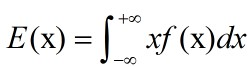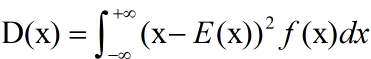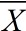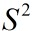# CTR预估中的贝叶斯平滑方法（二）参数估计和代码实现

## 1. 前言## 2. 参数估计的几种方法

### 1. 矩估计

1）根据给出的概率密度函数，计算总体的原点矩（如果只有一个参数只要计算一阶原点矩，如果有两个参数要计算一阶和二阶）。由于有参数这里得到的都是带有参数的式子。比如，有两个参数时，需要先计算出：期望; 方差。在Beta分布中，可以计算得到，E(x) = α / (α+β)，D(x) = αβ / (α+β)2(α+β+1)。

2）根据给出的样本，按照计算样本的原点矩。通常它的均值mean用表示，方差var用表示。（另外提一句，求时，通常用n-1为底。这样是想让结果跟接近总体的方差，又称为无偏估计）

3）让总体的原点矩与样本的原点矩相等，解出参数。所得结果即为参数的矩估计值。这里有，mean = E(x) = α / (α+β)，var = D(x) = αβ / (α+β)2(α+β+1)。于是乎，我们可以求得α，β：

α = [mean*(1-mean)/var - 1] * mean

β = [mean*(1-mean)/var - 1] * (1-mean)

### 2. Fixed-point iteration

1）首先给出参数的一个初始值（通常可以使用矩估计得到的结果作为初始值）。

2）在初始值处，构造似然函数的一个紧的下界函数。这个下界函数可以求得其最大值处的闭式解，将此解作为新的估计用于下一次迭代中。

3）不断重复上述（2）的步骤，直至收敛。此时便可到达似然函数的stationary point。如果似然函数是convex的，那么此时就是唯一的最优解。

1）2）由此，每次迭代，参数都会达到此次下界函数的最大值处，同时也就使得对应的似然函数值也相应地不断增大，直至收敛到似然函数的最大值处。

### 3. EM

（1）E-step：计算出隐含变量p在已观测数据（观测到的每个类别发生的次数，以及每个类别的超参数值的上一轮迭代的取值）下的后验分布，便可以得到完全数据的对数似然函数的期望值。

（2）M-step：对E-step中的期望值求最大值，便可得到相应的超参数的本轮迭代的更新值。

（3）不断重复地运行E-step和M-step，直至收敛。## 3. 代码实现

 1 #!/usr/bin/python
2 # coding=utf-8
3
4 import numpy
5 import random
6 import scipy.special as special
7 import math
8 from math import log
9
10
11 class HyperParam(object):
12     def __init__(self, alpha, beta):
13         self.alpha = alpha
14         self.beta = beta
15
16     def sample_from_beta(self, alpha, beta, num, imp_upperbound):
17         sample = numpy.random.beta(alpha, beta, num)
18         I = []
19         C = []
20         for click_ratio in sample:
21             imp = random.random() * imp_upperbound
22             #imp = imp_upperbound
23             click = imp * click_ratio
24             I.append(imp)
25             C.append(click)
26         return I, C
27
28     def update_from_data_by_FPI(self, tries, success, iter_num, epsilon):
29         '''estimate alpha, beta using fixed point iteration'''
30         for i in range(iter_num):
31             new_alpha, new_beta = self.__fixed_point_iteration(tries, success, self.alpha, self.beta)
32             if abs(new_alpha-self.alpha)<epsilon and abs(new_beta-self.beta)<epsilon:
33                 break
34             self.alpha = new_alpha
35             self.beta = new_beta
36
37     def __fixed_point_iteration(self, tries, success, alpha, beta):
38         '''fixed point iteration'''
39         sumfenzialpha = 0.0
40         sumfenzibeta = 0.0
41         sumfenmu = 0.0
42         for i in range(len(tries)):
43             sumfenzialpha += (special.digamma(success[i]+alpha) - special.digamma(alpha))
44             sumfenzibeta += (special.digamma(tries[i]-success[i]+beta) - special.digamma(beta))
45             sumfenmu += (special.digamma(tries[i]+alpha+beta) - special.digamma(alpha+beta))
46
47         return alpha*(sumfenzialpha/sumfenmu), beta*(sumfenzibeta/sumfenmu)
48
49     def update_from_data_by_moment(self, tries, success):
50         '''estimate alpha, beta using moment estimation'''
51         mean, var = self.__compute_moment(tries, success)
52         #print 'mean and variance: ', mean, var
53         #self.alpha = mean*(mean*(1-mean)/(var+0.000001)-1)
54         self.alpha = (mean+0.000001) * ((mean+0.000001) * (1.000001 - mean) / (var+0.000001) - 1)
55         #self.beta = (1-mean)*(mean*(1-mean)/(var+0.000001)-1)
56         self.beta = (1.000001 - mean) * ((mean+0.000001) * (1.000001 - mean) / (var+0.000001) - 1)
57
58     def __compute_moment(self, tries, success):
59         '''moment estimation'''
60         ctr_list = []
61         var = 0.0
62         for i in range(len(tries)):
63             ctr_list.append(float(success[i])/tries[i])
64         mean = sum(ctr_list)/len(ctr_list)
65         for ctr in ctr_list:
66             var += pow(ctr-mean, 2)
67
68         return mean, var/(len(ctr_list)-1)
69
70
71
72 def test():
73     hyper = HyperParam(1, 1)
74     #--------sample training data--------
75     I, C = hyper.sample_from_beta(10, 1000, 10000, 1000)
76     print I, C
77
78     #--------estimate parameter using fixed-point iteration--------
79     hyper.update_from_data_by_FPI(I, C, 1000, 0.00000001)
80     print hyper.alpha, hyper.beta
81
82     #--------estimate parameter using moment estimation--------
83     hyper.update_from_data_by_moment(I, C)
84     print hyper.alpha, hyper.beta

## 4. 参考文献

1. Click-Through Rate Estimation for Rare Events in Online Advertising

2. Estimating a Dirichlet distribution

本文由笨兔勿应所有，发布于http://www.cnblogs.com/bentuwuying。如果转载，请注明出处，在未经作者同意下将本文用于商业用途，将追究其法律责任。

posted @ 2017-03-04 12:21  笨兔勿应  阅读(7104)  评论(5编辑  收藏  举报